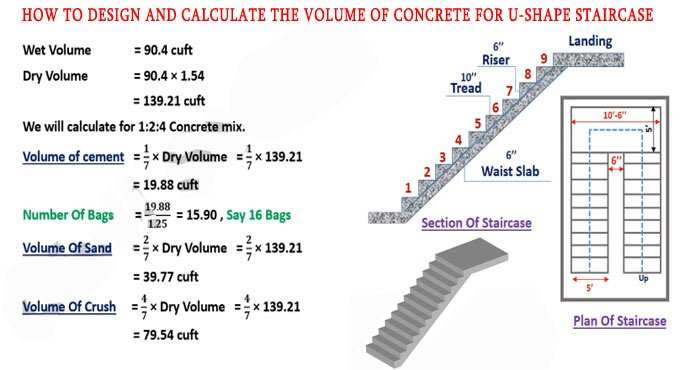Breaking News
Home / Civil Engineering / Volume Calculation Of Concrete Staircase

# Volume Calculation Of Concrete Staircase

## Volume Calculation of Concrete Staircase

The maximum riser of the staircase should be 7 inches and a minimum of 4 inches. The minimum tread should be 10 inches and a maximum of 12 inches or 1 feet. The breadth of the stair should be 5 feet. The landing of the stair should be a minimum of 10 feet 6 inches. The spacing among two flights should be a minimum of 6 inches. The steps in the stair range from 1 to 9. The waist thickness of the slab should be a minimum of 6 inches.

The calculation is done on the basis of the following dimensions:-

Height of floor = 10 feet

Height of one flight = 10/2 = 5 feet

Height of Risers = 6 inches = 0.5 feet

Number of risers = Height of flight/Riser = 5/0.5 = 10 nos.

So, total steps along with landing = 10 nos.

Length of tread = 10 inches = 0.8 feet

Number of treads = (Number of risers – 1) = 10 – 1 = 9 nos.

Thickness of waist slab = 6 inches = 0.5 feet

Length of step = 5 feet

With the use of the following formula, the volume of concrete will be determined for steps:-

Volume of one step = 1/2 x riser x tread x length of step

### Difference Between Plinth Level, Ground Level, Sill Level and Slab Level

After putting all the values, we get the following:-

1/2 x 0.5 x 0.83 x 5 = 1.03 cubic feet

As there are 9 number of steps in a flight, so the volume of steps for first flight = 9 x 1.03 = 9.27 cubic feet

Now, it is required to determine the concrete volume for the waist slab. Before that, you have to find out the inclined length of the waist slab by applying the following formula:-

√(horizontal length)2 + (height)2

But, the horizontal length & height is unknown here.

The horizontal length will be computed as follow:-

The horizontal length of waist slab = Tread x Number of Steps

After putting the values, we get the following:-

0.8333 X 9 = 7.4 feet

Now, it is required to determine the height of the top of the landing from floor as follow:-

Number of riser x Height of riser = 10 x 0.5 = 5 feet

So, inclined length of waist slab = √(horizontal length)2 + (height)2

= √(7.4)2 + (5)2 = √79.96 = 8.93 feet### THANKS.